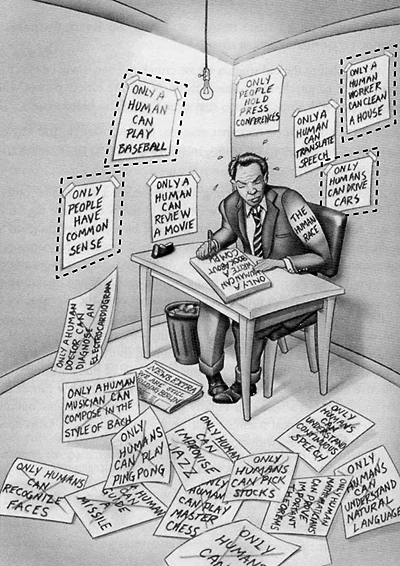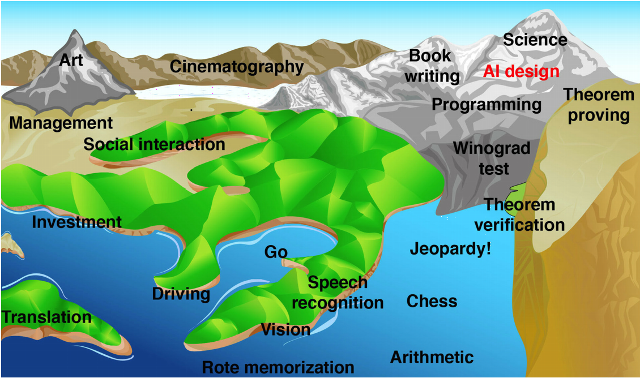# Guiding Human Intuition in Pure Mathematics with Machine Learning

A paper published on 2021-12-01 in Nature, “Advancing mathematics by guiding human intuition with AI” (full text [PDF]), reports work by DeepMind on using machine learning to assist pure mathematicians in formulating conjectures, long considered a central part of the creative process in mathematical research. Here is the abstract.

The practice of mathematics involves discovering patterns and using these to formulate and prove conjectures, resulting in theorems. Since the 1960s, mathematicians have used computers to assist in the discovery of patterns and formulation of conjectures, most famously in the Birch and Swinnerton-Dyer conjecture, a Millennium Prize Problem. Here we provide examples of new fundamental results in pure mathematics that have been discovered with the assistance of machine learning—demonstrating a method by which machine learning can aid mathematicians in discovering new conjectures and theorems. We propose a process of using machine learning to discover potential patterns and relations between mathematical objects, understanding them with attribution techniques and using these observations to guide intuition and propose conjectures. We outline this machine-learning-guided framework and demonstrate its successful application to current research questions in distinct areas of pure mathematics, in each case showing how it led to meaningful mathematical contributions on important open problems: a new connection between the algebraic and geometric structure of knots, and a candidate algorithm predicted by the combinatorial invariance conjecture for symmetric groups. Our work may serve as a model for collaboration between the fields of mathematics and artificial intelligence (AI) that can achieve surprising results by leveraging the respective strengths of mathematicians and machine learning.

I guess it’s time to drag out this image once again……and this one.1 Like

Understanding mathematics and machine learning are way beyond me. My only solace is that my son is able to understand, having earned an MS in math and a Ph.D. in Genetics/Bioinformatics by performing GWAS (genome-wide association studies), which rely heavily on sophisticated statistical, mathematical and modeling constructs. I like to think that maybe, to a small extent at least, he ‘stood on my shoulders’ intellectually and can see far more of the horizon than can I. Such is the only existential solace for those of us who are unable to believe in the traditional religious sense.

1 Like